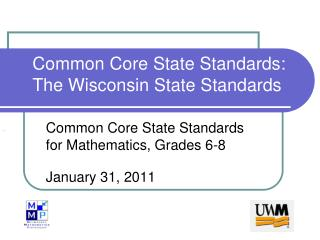DownloadDownload PresentationCommon Core State Standards: The Wisconsin State Standards

# Common Core State Standards: The Wisconsin State Standards

Télécharger la présentation## Common Core State Standards: The Wisconsin State Standards

- - - - - - - - - - - - - - - - - - - - - - - - - - - E N D - - - - - - - - - - - - - - - - - - - - - - - - - - -
##### Presentation Transcript

1. Common Core State Standards: The Wisconsin State Standards Common Core State Standards for Mathematics, Grades 6-8 January 31, 2011

2. Learning Intentions We are learning to: • recognize the first of the Standards for Mathematical Practice within a chosen Content Standard. • identify those standards within a particular math task.

3. Success Criteria We will know we are successful when we can articulate how both a Content Standard and a Standard for Mathematical Practice are infused in a math lesson in the classroom.

4. Vocabulary • Domain: Ratios and Proportional Relationships (6.RP) • Cluster: Understand ratio concepts and use ratio reasoning to solve problems. • Standard 3: Use ratio and rate reasoning to solve real-world and mathematical problems, e.g., by reasoning about tables of equivalent ratios, tape diagrams, double number line diagrams, or equations. (See standard for specific examples)

5. Unpacking the Content Standard Individually read Standard 3 ? – “I have a question about this.” * - “This makes sense to me.” With your group, discuss questions and highlight key ideas in this standard.

6. Content Standard Discussion Standard 3: Use ratio and rate reasoning to solve real-world and mathematical problems, e.g., by reasoning about tables of equivalent ratios, tape diagrams, double number line diagrams, or equations.

7. Unpacking the Practice Standard 1. Make sense of problems and persevere in solving them. Individually read the practice standard ? – “I have a question about this.” * - “This makes sense to me.” With your group, discuss questions and highlight key ideas in this standard.

8. Linking Practice to the Classroom • What would this practice look like in a classroom? • Students would be… • Teachers would be…

9. Chocolate and Cream A candy factory has a large vat into which workers pour chocolate and cream. Each ingredient flows into the vat from its own special hose, and each ingredient comes out of its hose at a constant rate. Workers at the factory know that it takes 20 minutes to fill the vat with chocolate from the chocolate hose, and it takes 15 minutes to fill the vat with cream from the cream hose. If workers pour both chocolate and cream into the vat at the same time (each coming full tilt out of its own hose), how long will it take to fill the vat? Before you find an exact answer to this problem, find an approximate answer, or find a range, such as “between…and…minutes.” Explain your reasoning.

10. What did we see? • What did you as students do to bring the standards alive? • What did the facilitator as a teacher do to bring the standards alive? • What else might you as teachers in your classroom do to bring the standards alive?

11. WI meets CCSS

12. Connections • Identify other Standards for Mathematical Practice that you saw as we carried out today’s task. • Use specific examples to explain the connection between the content and practice standard you identified.

13. Summary We are learning to recognize the first of the Standards for Mathematical Practice within a chosen Content Standard and identify those standards within a particular math task. We will know we are successful when we can articulate how both a Content Standard and a Standard for Mathematical Practice are infused in a math lesson in the classroom.